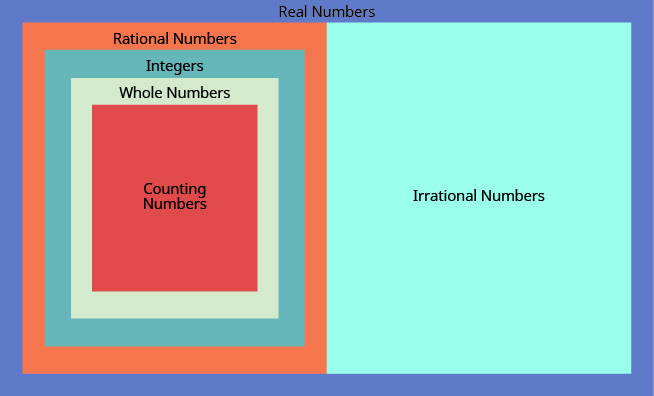# CHAPTER 2 Operations with Rational Numbers and Introduction to Real Numbers

All the numbers we use in the intermediate algebra course are real numbers. The chart below shows us how the number sets we use in algebra fit together. In this chapter we will work with rational numbers, but you will be also introduced to irrational numbers. The set of rational numbers together with the set of irrational numbers make up the set of real numbers.